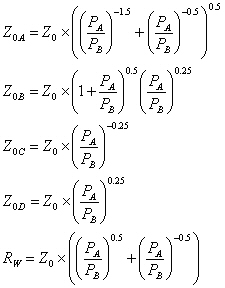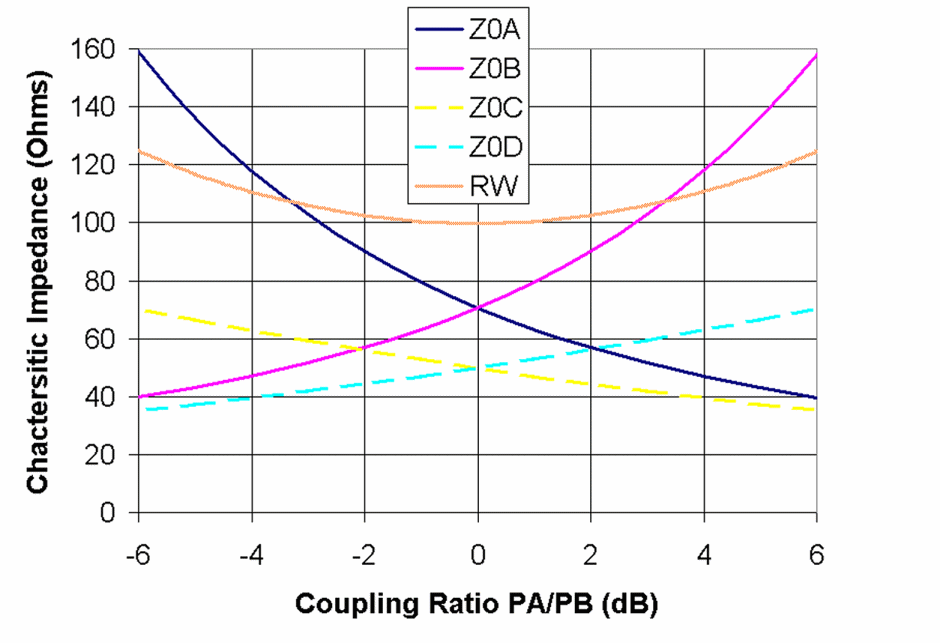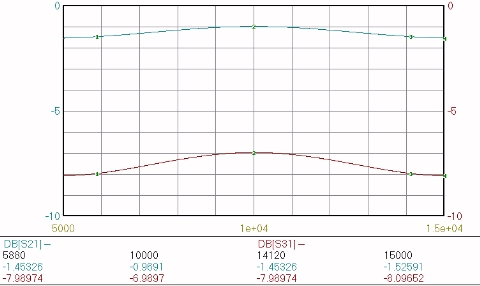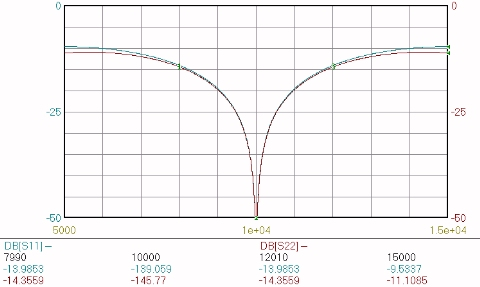# Split Tee Power Divider

Click here to go to our page first page on unequal Wilkinson splitters

This page looks at the power divider first described in 1965 by Lenny Parad and Robert Moynihan in their IEEE paper Split Tee Power Divider. The split-tee is really an unequal split Wilkinson, but Split Tee is what the author's named it. As we'll show you on other pages of this web site, there are more than one solution to creating an unequal split Wilkinson, but they all should be compared to the original method described by Parad and Moynihan.

We have an in-depth look at the split tee power divider on this page.

Almost all microwave textbooks, and many professional papers reference the split tee power divider, or use the equations from it without referencing the source. Heck, some papers reference textbooks that reference the paper, the origin of the equations is getting lost! One patent that references the split tee power divider is Cliff Quan's attenuator. It's too bad they spelled Moynihan "Moyniham!"

The Split Tee paper describes two networks, one that uses four quarterwave sections, and another that uses five (a transformer is used on the input to make the other section impedances more realizable). For now we'll describe the four-section network only.

The equations from Split Tee Power Divider are used in our unequal split Wilkinson on-line calculator.

The source for the unequal-split Wilkinson equations is an IEEE article from 1965 by Parad and Moynihan, entitled Split Tee Power Divider, published in IEEE Transactions on Microwave Theory and Techniques in January, 1965. There is a second example in Parad and Moynihan's paper which adds an input transformer, if you prowl around the world wide web you will find information about it (one of these days we'll include it on this page).

The following set of equations when satisfied ensure that the ports will be matched and port 2 and port 3 will be isolated. These are Parad and Moynihan's equations, and have been cited in all of the best microwave text books like Pozar's.Plotted below are the four line impedances and the isolation resistor value as functions of the power split express in dB.Line impedances and isolation resistor for unequal-split Wilkinson

Below are plots of the power split and impedance match for PA/PB=4.0. The insertion loss bandwidth is reduced compared to the equal-split Wilkinson, but the isolation bandwidth is nearly the same. We didn't bother to plot the isolation.Unequal-split Wilkinson, PA/PB=4.0Unequal-split Wilkinson, PA/PB=4.0